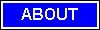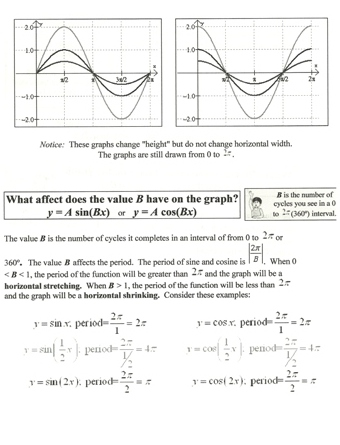M A T H   T U T O R I N G
ONE-ON-ONE
TEST PREPARATION917.392.7004    email: mathtutor255@yahoo.com

Trigonometry is a branch of mathematics that studies triangles and the relationships between their sides and the angles between sides. Trigonometry defines the trigonometric functions,Radian,Sines, Cosines, Tangens, which describe those relationships and have applicability to cyclical phenomena, such as waves. The field evolved during the third century BC as a branch of geometry used extensively for astronomical studies. If one angle of a triangle is 90 degrees and one of the other angles is known, the third is thereby fixed, because the three angles of any triangle add up to 180 degrees. The two acute angles therefore add up to 90 degrees: they are complementary angles. The shape of a triangle is completely determined, except for similarity, by the angles. Once the angles are known, the ratios of the sides are determined, regardless of the overall size of the triangle.sitemap